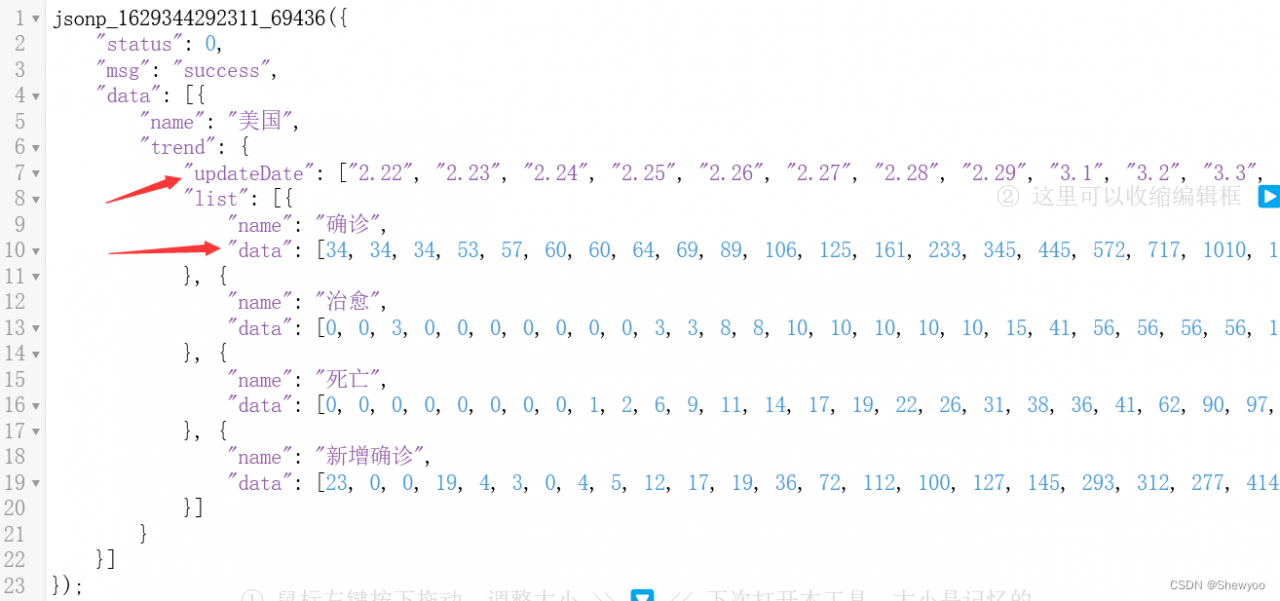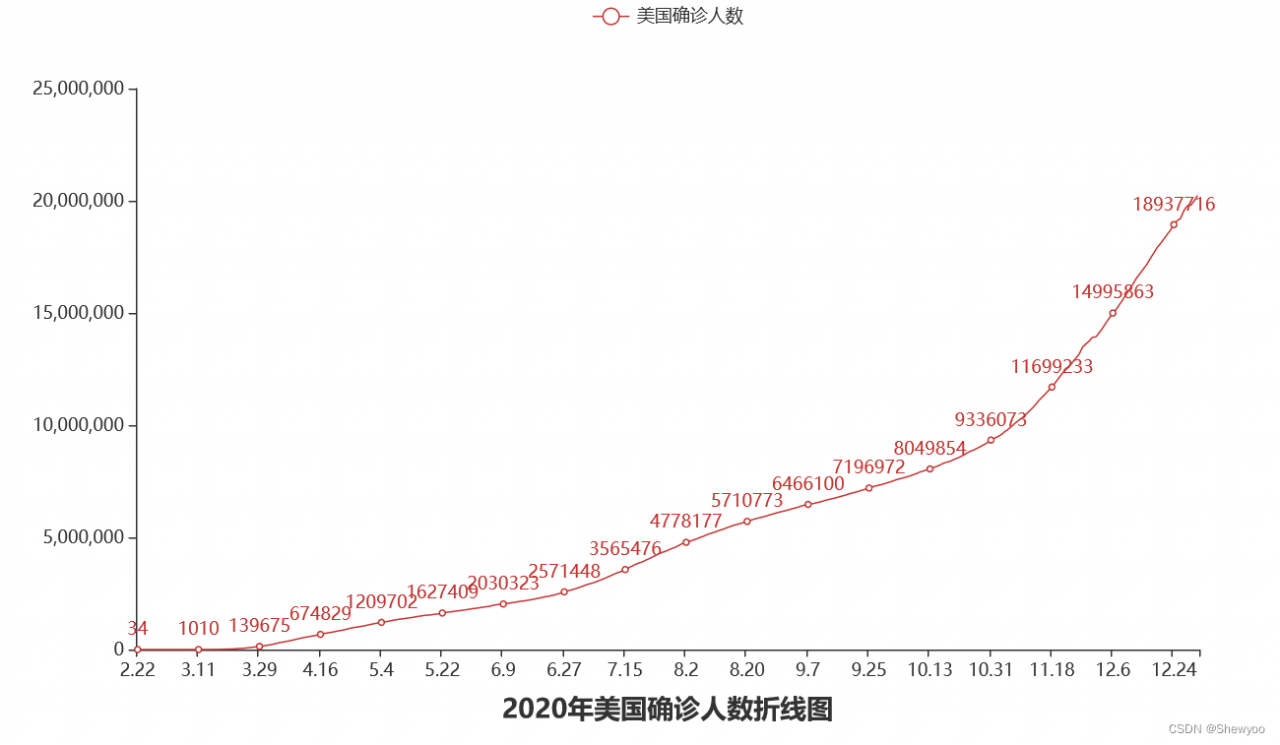# 【Python】– 数据可视化案例（疫情确诊的折线图）

### 案例描述# 实现步骤

## 一、导入模块

``````import json
from pyecharts.charts import Line``````

## 二、读取文件内容

``````f_us = open("D:/美国.txt","r",encoding="UTF-8")

### 去掉不符合json规范的开头

json文件是一个单独的字典或一个内部元素都是字典的列表。

``us_data = us_data.replace("jsonp_1629344292311_69436(","")``

### 去掉不符合json规范的结尾

``us_data = us_data[:-2]``

## 三、json转换python

``us_dict = json.loads(us_data)``

## 四、获取需要用到的数据

``````# 获取trend key
trend_data = us_dict['data']['trend']
# 获取日期数据，取2020一年的数据,到314下标结束
x_data = trend_data['updateDate'][:314]
# 获取确诊数据
y_data = trend_data['list']['data'][:314]``````

## 五、生成图表

### 构建对象

``line = Line()``

### 添加x、y轴数据

``````line.add_xaxis(x_data)

``line.add_yaxis("美国确诊人数",y_data,label_opts=LabelOpts(is_show=False))``

### 设置全局选项

``````# 先导入需要用到的模块
from pyecharts.options import TitleOpts
# 设置
line.set_global_opts(
title_opts=TitleOpts(title="2020年美国确诊人数折线图",pos_left="center",pos_bottom="1%")
)``````

### 调用render方法，生成图表

``line.render()``

## 六、关闭文件

``f_us.close()``